Author: Oscar Cronquist Article last updated on August 05, 2021

####Use the new XLOOKUP function which is an improved version of the HLOOKUP function available for Excel 365 users.

## 1. HLOOKUP function - Overview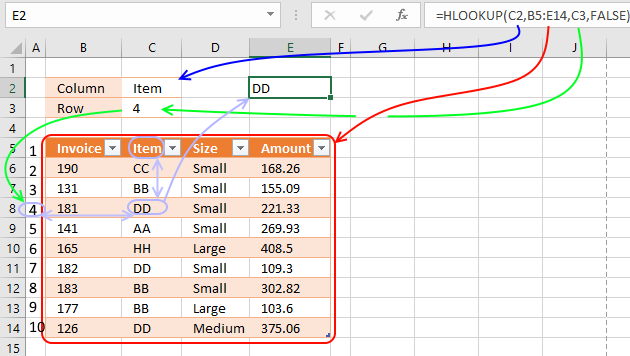The HLOOKUP function lets you search the top row in a data range for a value and return another value on the same column in a row you specify.

HLOOKUP stands for horizontal lookup and your data (table_array) must be organized into records, a record on each row.

To search records excel must look horizontally in the top row of the data array. VLOOKUP stands for vertical lookup but that is another post.

Formula in cell E2:

=HLOOKUP(C2,B5:E14,C3,FALSE)

The HLOOKUP function in cell E2 searches the top row of cell range B5:E14 for the value "Item" and it is found in the second column.

The third argument tells the function which row to fetch the value from, in this case, it is four.

The intersection of the second column and the fourth row is value DD and that is the returning value in cell E2.

The HLOOKUP function is designed to return only one value, however, there are workarounds to fetch all matching values.
5 easy ways to VLOOKUP and return multiple values
I recommend the FILTER function if you are an Excel 365 user.

## 2. HLOOKUP Function Syntax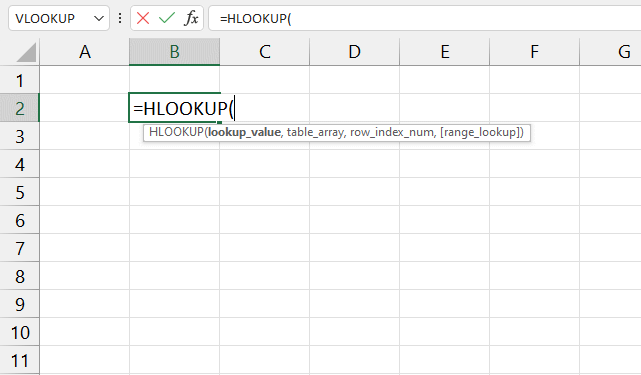HLOOKUP(lookup_value, table_array, col_index_num, [range_lookup])

## 3. HLOOKUP Function Arguments

 Lookup_value Required. The first argument is the lookup value. This is the value you want to find in the top row of the table array. Table_array Required. This is a cell range or an array that you want to search. Row_index_num Required. Here you specify which row you want fetch the value from. Range_lookup Optional. TRUE tells the function to do an approximate match and this is the default value. FALSE - Exact match

Make sure the top row in the table array is sorted in ascending order. FALSE means exact match and this mode is probably what you will use the most. Take note that if you use the wrong argument here you may get incorrect results.

## 5. Approximate match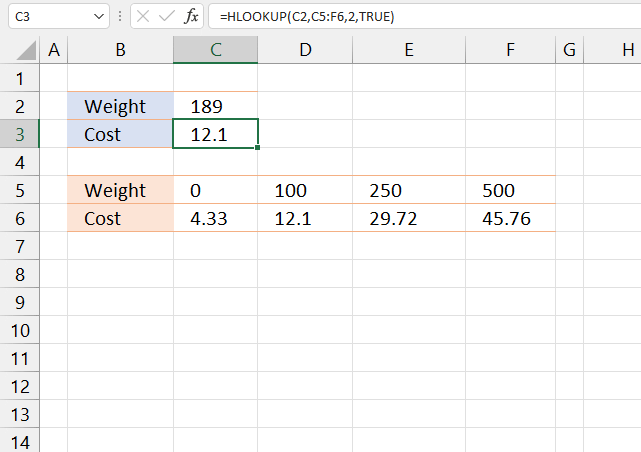The HLOOKUP function lets you search using an approximate match if you enter TRUE in the fourth argument or don't specify anything at all. In the example above there are four different ranges, 0 and less than 100, 100 and less than 250, 250 and less than 500, and 500 and more.

The approximate match setting finds the largest value that is less than or equal to the lookup_value.

The value 189 in cell C2 can't be found in the top row of cell range C5:F6 so the largest value that is less than lookup_value (189) is matched, in this case, 100. The corresponding value is 12.1 and that value is returned in cell C3.

You want, in most cases, to use the EXACT match, however, the default value is an APPROXIMATE match. So make sure you know what you are doing.

True - Approximate match (default)
False - Exact match

This means that you get the approximate match if you don't specify the fourth argument at all. It is very important that the top row is sorted in ascending order if the fourth argument is left out or is True (approximate match).

## 6. Horizontal and vertical lookup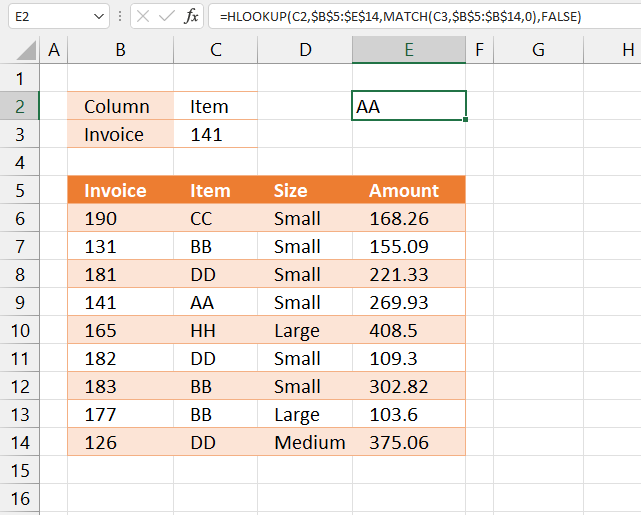The following formula lets you search both horizontally and vertically. The formula in cell E2 looks for Item in the top horizontal row and 141 in column "Invoice", the intersecting cell is C9 so the formula returns AA.

This example demonstrates a formula that combines both the MATCH and HLOOKUP functions to perform a horizontal and vertical lookup.

Formula in cell E2:

=HLOOKUP(C2,B5:E14,MATCH(C3,B5:B14,0),FALSE)

### Explaining formula in cell E2

#### Step 1 - Find the relative position

The MATCH function returns the relative position of an item in an array or cell reference that matches a specified value in a specific order.

MATCH(lookup_value, lookup_array, [match_type])

MATCH(C3, B5:B14, 0)

The MATCH function returns a number representing the row of which a given value is found.

MATCH(C3, B5:B14, 0)

becomes

MATCH(141, {"Invoice"; 190; 131; 181; 141; 165; 182; 183; 177; 126}, 0)

and returns 5. Number 141 is the fifth position in B5:B14.

#### Step 2 - Find value and return corresponding value based on the row number

HLOOKUP(C2, B5:E14, MATCH(C3, B5:B14, 0), FALSE)

becomes

HLOOKUP(C2, B5:E14, 5, FALSE)

and returns "AA".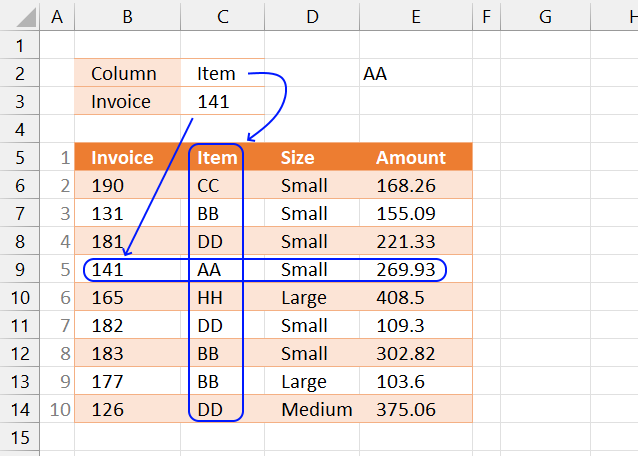## 7. Horizontal and vertical lookup - INDEX + MATCH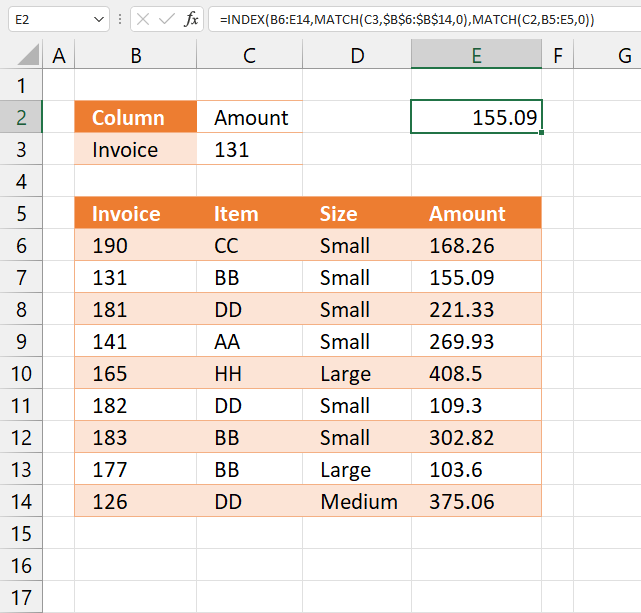You can also use INDEX and MATCH functions to get a value in a cross-reference table. The formula below works just like the formula above in section 6.

Formula in cell E2:

=INDEX(B6:E14, MATCH(C3, B6:B14, 0), MATCH(C2, B5:E5, 0))

This formula is actually easier to understand and remember than the HLOOKUP + MATCH functions, this is the method I prefer.

### Explaining formula in cell E2

#### Step 1 - Find the relative position of the column header name

The MATCH function returns the relative position of an item in an array or cell reference that matches a specified value in a specific order.

MATCH(lookup_value, lookup_array, [match_type])

MATCH(C2,B5:E5,0)

becomes

MATCH("Amount",{"Invoice","Item","Size","Amount"},0)

and returns 4. "Amount" is the fourth value in the array.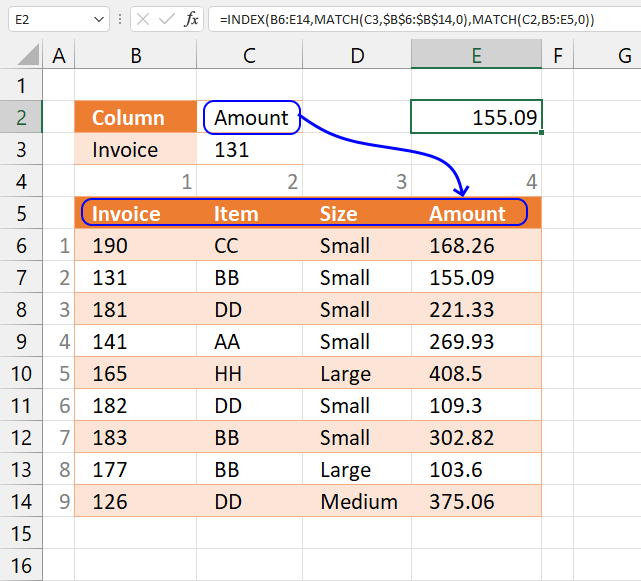#### Step 2 - Find the relative position vertically

MATCH(C3, B6:B14, 0)

becomes

MATCH(131,{190; 131; 181; 141; 165; 182; 183; 177; 126},0)

and returns 2. 131 is the second value in the array.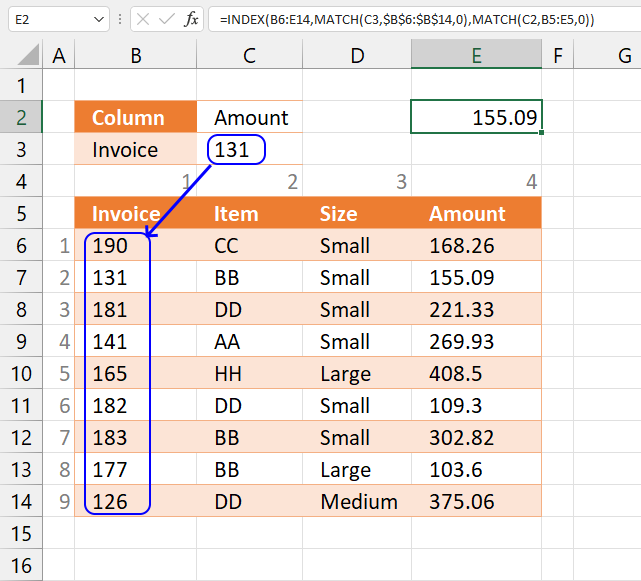#### Step 3 - Get value based on row and column number

The INDEX function returns a value based on a row and column number.

INDEX(array, [row_num], [column_num])

INDEX(B6:E14, MATCH(C3, B6:B14, 0), MATCH(C2, B5:E5, 0))

becomes

INDEX(B6:E14, 2, 4)

and returns 155.09 in cell E2.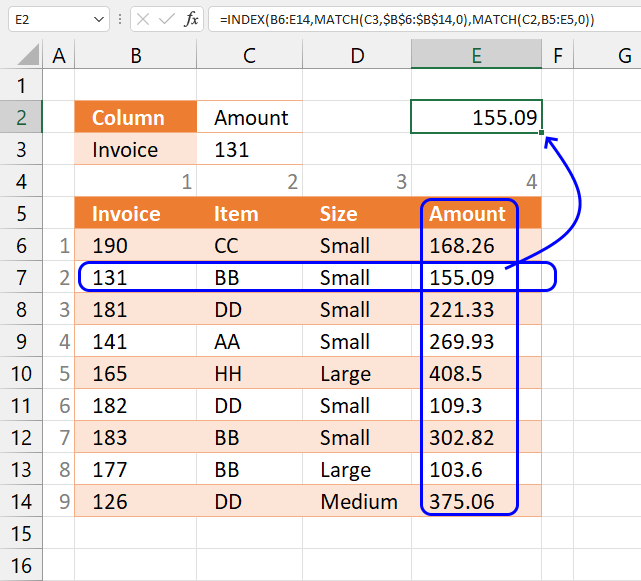## 8. HLOOKUP - multiple tables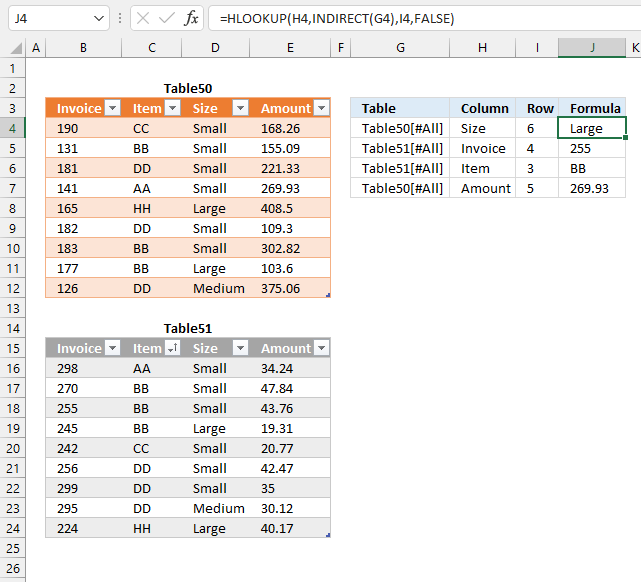The formula below uses a text string specified in a cell to search a given Excel Table using a lookup value.

There are two Excel Tables in the image above, Table50 and Table51. Column G contains which Excel Table to searc, column H which column to search, and finally column I which row.

Formula in cell J4:

=HLOOKUP(H4, INDIRECT(G4), I4, FALSE)

### Explaining formula in cell J4

#### Step 1 - Convert text string to a structured reference

The INDIRECT function lets you convert a cell value to a cell reference or in this case a structured reference.

The INDIRECT function is volatile, so use it with care. Volatile means it is recalculated every time the worksheet is recalculated. If you have many INDIRECT functions, they may slow down your workbook considerably.

INDIRECT(G4)

becomes

INDIRECT("Table50[#All]")

and returns Table50[#All].

#### Step 2 - Get value from cell range

HLOOKUP(H4, INDIRECT(G4), I4, FALSE)

becomes

HLOOKUP("Size", Table50[#All], 6, FALSE)

and returns "Large" in cell J4.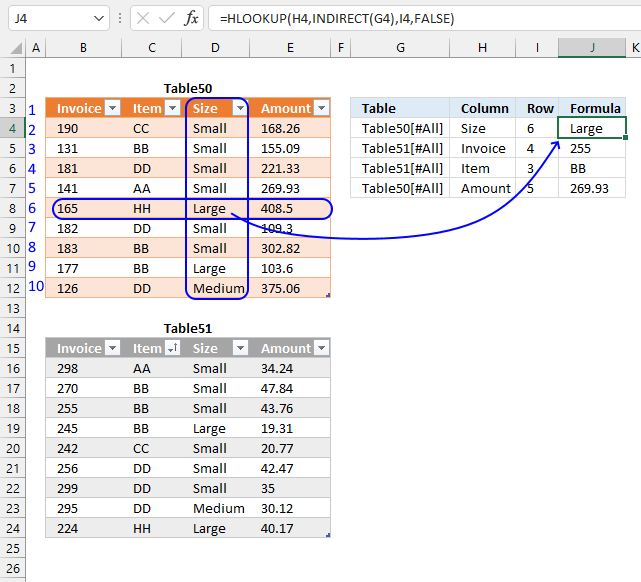## 9. HLOOKUP - VBA Example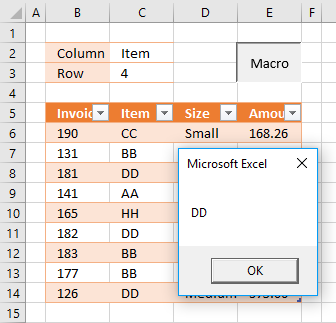The following macro lets you search a cell range using the parameters specified in cells C2 and C3. A messagebox appears and tells you the found value.

VBA Code

```Sub HLP()
MsgBox Application.WorksheetFunction.HLookup(Range("C2"), Range("B5:E14"), Range("C3"), False)
End Sub
```